# Find the resistance R in this network

• Engineering
Edy56
Homework Statement:
I am supposed to find the equivalent to R2 and I got 2, but I am supposed to get 3
Relevant Equations:
None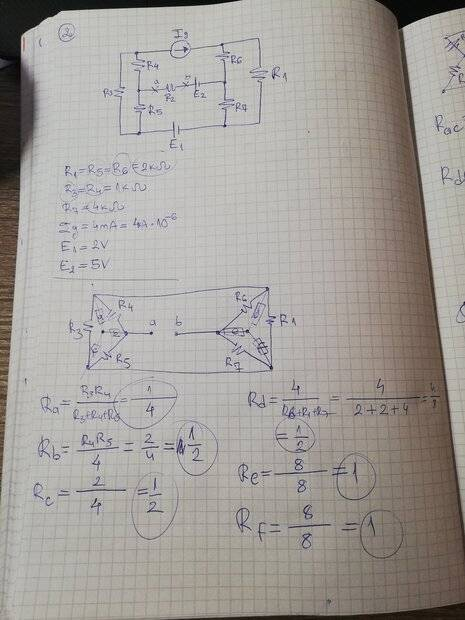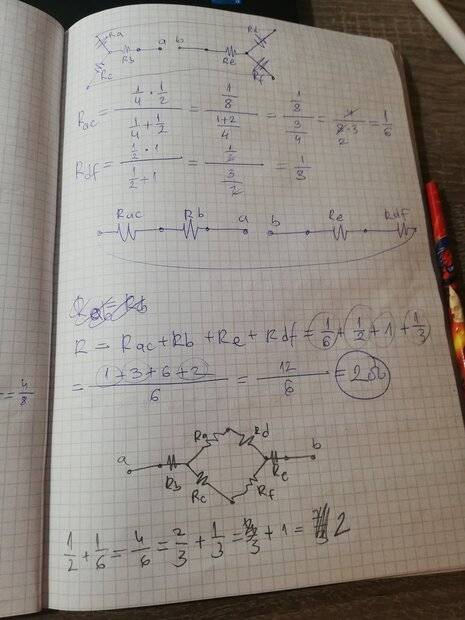Mentor
Welcome to PF.It's pretty hard for me to read your uploaded pictures. Can you post a better picture of the original circuit, and type some of your work into the forum so it's readable? Thanks.

(you should consider learning to post math using LaTeX -- see the "LaTeX Guide" link below the Edit window)

Gold Member
2022 Award
It's pretty hard for me to read your uploaded pictures. Can you post a better picture of the original circuit, and type some of your work into the forum so it's readable? Thanks.Edy56
Welcome to PF.It's pretty hard for me to read your uploaded pictures. Can you post a better picture of the original circuit, and type some of your work into the forum so it's readable? Thanks.

(you should consider learning to post math using LaTeX -- see the "LaTeX Guide" link below the Edit window)
the circuit: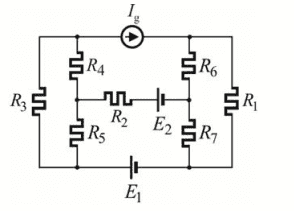here is what I did:
(english is not my first language so sorry if i get any terms wrong)
I thought R3, R4 and R5 form a triangle so I turned it into a star for easier calculation and I did the same for R6 R7 and R1.
Then I thought R34 and R35 are parallel so I calculated that too and the same I did for R61 and R71.
Then I thought all of them were connected in order meaning I could add them all together.
That is:
R3435 +R45 + R67 + R6171.
I got the answer 2, I need to get 3.
Where did I go wrong?

Gold Member
2022 Award
How could we possibly know where you went wrong when you have not shown your work ???

SHOW EVERY STEP

Edy56
How could we possibly know where you went wrong when you have not shown your work ???

SHOW EVERY STEP
It's at the beginning of the thread

Gold Member
2022 Award
It's at the beginning of the thread
Yes, and it got this response:
It's pretty hard for me to read your uploaded pictures. Can you post a better picture of the original circuit, and type some of your work into the forum so it's readable? Thanks.

Edy56
the circuit:
View attachment 321978
here is what I did:
(english is not my first language so sorry if i get any terms wrong)
I thought R3, R4 and R5 form a triangle so I turned it into a star for easier calculation and I did the same for R6 R7 and R1.
Then I thought R34 and R35 are parallel so I calculated that too and the same I did for R61 and R71.
Then I thought all of them were connected in order meaning I could add them all together.
That is:
R3435 +R45 + R67 + R6171.
I got the answer 2, I need to get 3.
Where did I go wrong?
That's what I did here?

Gold Member
2022 Award
That's what I did here?
No, you listed the steps you did not show any of the calculations.

THIS:
"I thought R3, R4 and R5 form a triangle so I turned it into a star for easier calculation and I did the same for R6 R7 and R1."

is a set of steps. It is not calculations.

Edy56
No, you listed the steps you did not show any of the calculations.

THIS:
"I thought R3, R4 and R5 form a triangle so I turned it into a star for easier calculation and I did the same for R6 R7 and R1."

is a set of steps. It is not calculations.
I am not sure as to how write that. I can draw it, but I don't know how to write those steps.

If you mean calculating new Rs it's just basic multiplication and I have in the picture.

Gold Member
2022 Award
I'm sure you could figure out how to type numbers and equations, but I can see that it would be a lot of work. Perhaps someone else here will be willing to look at your hand-written work and follow it to see if they can find your error.

Edy56
I'm sure you could figure out how to type numbers and equations, but I can see that it would be a lot of work. Perhaps someone else here will be willing to look at your hand-written work and follow it to see if they can find your error.
Okay

I cannot read the component values.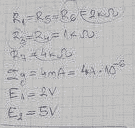Edy56
I cannot read the component values.
View attachment 321988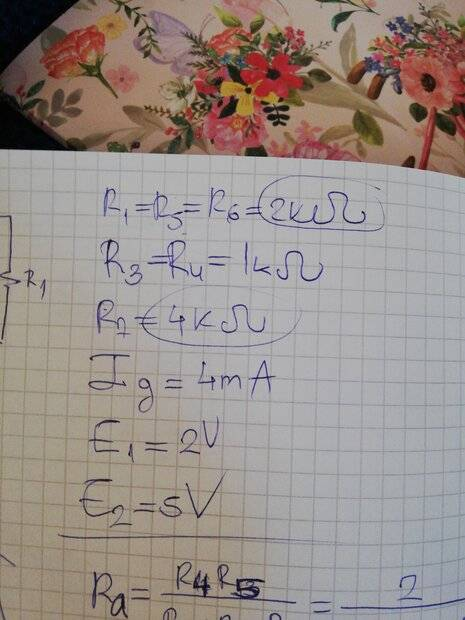You do not need the Y to Δ transform.
Current source Ig is infinite resistance, so remove it from the circuit.
Voltage sources, E1 and E2 are zero resistance, so short them.
R1 = R5 = R6 = 2k;
R3 = R4 = 1k;
R7 = 4k.
Series; +
Parallel; //
R16 = R1 + R6 =
R34 = R3 + R4 =
R167 = R16 // R7 =
R345 = R34 // R5 =
R2 = R167 + R345 = 3k.

Edy56
You do not need the Y to Δ transform.
Current source Ig is infinite resistance, so remove it from the circuit.
Voltage sources, E1 and E2 are zero resistance, so short them.
R1 = R5 = R6 = 2k;
R3 = R4 = 1k;
R7 = 4k.
Series; +
Parallel; //
R16 = R1 + R6 =
R34 = R3 + R4 =
R167 = R16 // R7 =
R345 = R34 // R5 =
R2 = R167 + R345 = 3k.
I am not sure I understand...
The way I do it, I imagine that power is flowing from a to b and so when it's going from a the power splits in two because of R4 and R5 and thus making them parallel. Why isn't it like that?